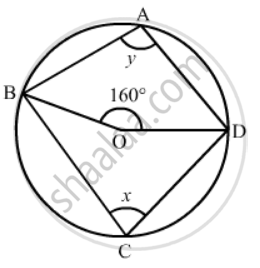Advertisement Remove all ads

# In the Given Figure, O is the Centre of the Circle. If ∠Bod = 160°, Find the Values of X and Y. - Mathematics

Short Note

In the given figure, O is the centre of the circle. If ∠BOD = 160°, find the values of x and y.Advertisement Remove all ads

#### Solution

It is given that O is centre of the circle and BOD = 160°We have to find the values of and y.

As we know that the angle subtended by an arc of a circle at the centre is double the angle subtended by it at any point on the remaining part of the circle.

Therefore,

x = 1/2 (160°)

= 80°

Since, quadrilateral ABCD is a cyclic quadrilateral.

So,

x + y = 180°              (Sum of opposite angles of a cyclic quadrilateral is 180°.)

 y = 180°  - x

=180°  -  80°

= 100°

Hence angle = 100°  and   anglex = 80°

Is there an error in this question or solution?
Advertisement Remove all ads

#### APPEARS IN

RD Sharma Mathematics for Class 9
Chapter 15 Circles
Exercise 15.5 | Q 3 | Page 101
Advertisement Remove all ads
Advertisement Remove all ads
Share
Notifications

View all notifications

Forgot password?
Course